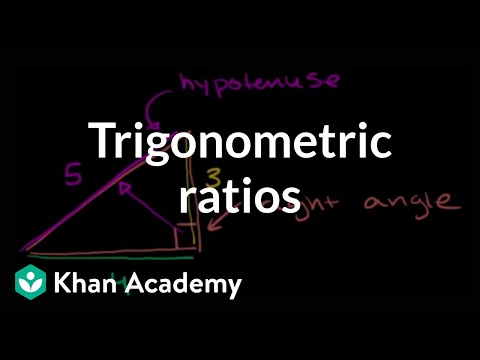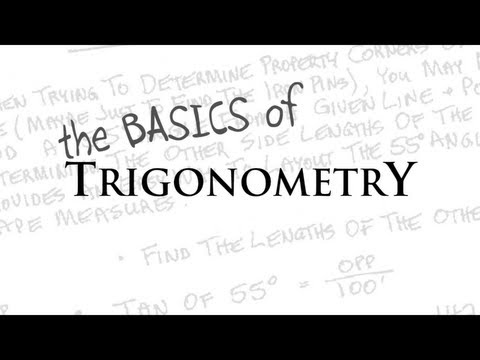## Trigonometry

Subject: Compulsory Maths

Find Your Query

#### Overview

The word trigonometry comes from the combination of the words "tri"and goes on having the meaning of the measurement of three angles of a triangle. With the help of right-angled triangle, trigonometric ratios of an angle are found. here we will calculate the values of trigonometric ratios as form special angles 0 degrees, 30 degrees, 45, 60 and 90 degrees etc.

##### Trigonometry

The word "Trigonometry" is derived from the Greek word "Tri-Gonia-Metron" where 'Tri' means 'three', 'Gonia' means 'angles' and 'Metron' mean 'measure'. So, trigonometry is a branch of mathematics which concerned with the measurement of sides, angles and their relation to a triangle.

#### Trigonometric RatiosThe word trigonometry comes from the combination of the word triangle goes on having the meaning of the measurement of three angles of triangles. Hence, this is also known as the measure of the triangle. Trigonometry has wide application in the field of mathematics and science. With the help of right angles triangle, trigonometric ratios of an angle are found. Without the help of trigonometric ratios, both the development and expansion of physics and also of engineering are impossible. Hence, trigonometry is the very important branch of mathematics.

Above figure shows the shadow of the poles formed at 3 pm that stand perpendicularly on the road. For each figure, the ratio of height of pole and length of shadow and height: length are tabulated below:

 Pole height of pole length of shadow height:length angle made with the ground a 3m 2m 3:2 56o b 6m 4m 3:2 56o

Hence, the height of every pole and the length of their shadows are in proportion. The angle made by the top of the pole and with the top of shadow on the ground is also equal.Trigonometric Ratios of some special angles#### Fundamentals of Trigonometric Ratios

The ratio of any two sides of a right-angled triangle taking one of the side as reference are the fundamentals of trigonometric ratios.Let know on detail about ratio with a figure. Here, in the given figure, ΔABC is a right angled triangle. $\angle$B = 90oand $\angle$C =θ.

Let's take$\angle$C as a reference angle

The opposite side of angle C (perpendicular) (P) = AB

The adjacent side of angle C (base) (B) = BC and (hypotenuse) (H) = AC

We can make three relation with the reference angle.

• relation between perpendicular and hypotenuse

In the above figure, the ratio of AB (perpendicular) to AC (base) with reference angle is called sine θ.

∴ sin θ = $\frac{AB}{AC}$ = $\frac{perpendicular}{hypotenuse}$ = $\frac{p}{h}$

• relation between base and hypotenuse

In the above figure, the ratio of BC (base) to AC (base) with reference angle is called cosine θ.

∴ cos θ = $\frac{BC}{AC}$ = $\frac{base}{hypotenuse}$ = $\frac{b}{h}$

• relation between perpendicular and base

In the above figure, the ratio of AB (perpendicular) to BC (base) with reference angle is called tangent θ.

∴ tan θ = $\frac{AB}{BC}$ = $\frac{perpendicular}{base}$ = $\frac{p}{b}$#### Pythagoras TheoremThe relationship between the three sides of a triangle is simply known as Pythagoras Theorem. The relation was given by the popular Mathematician Pythagoras so it is called as Pythagorean theorem.
In mathematics, the Pythagorean theorem, also known as Pythagoras's theorem, is a relation in Euclidean geometry among the three sides of a right triangle. It states that the square of the hypotenuse (the side opposite the right angle) is equal to the sum of the squares of the other two sides.
According to this theorem "In any right-angled triangle, the area of the square on the hypotenuse is equal to the sum of the areas of squares of perpendicular and base".

By Pythagoras Theorem

Hypotenuse (h2) = Perpendicular (p2) + Base (b2)
or, h2= p2+ b2
From this theory we can derive,
h = $\sqrt{p^{2} + b^{2}}$
p = $\sqrt{h^{2} - b^{2}}$
b = $\sqrt{h^{2} - p^{2}}$

Theoretical proof:

Given: ΔABC is a right angled triangle in which $\angle$ABC = 90o.

To prove: CA2 = AB2 + BC2

Construction: BD ⊥AC is draawn

Proof:

 S.N. Statements Reasons 1. In ΔABC and ΔBCD i. $\angle$ABC =$\angle$BDC (A) Both of them are right angles ii. $\angle$BCA =$\angle$BCD (A) ommon angles iii. $\angle$BAC = $\angle$DBC (A) Remaining angles of the triangles 2. $\frac{CA}{BC}$ = $\frac{BC}{CD}$ or, BC2 = CA.CD A.A.A. axiom 3. ΔABC∼ΔABD Same as above 4. $\frac{CA}{AB}$ = $\frac{AB}{AD}$ or, AB2 = CA.AD Corresponding sides of similar triangles 5. AB2 + BC2 = CA.AD + CA.CD or, AB2 + BC2 = CA (AD + CD) or, AB2 + BC2 = CA.CA or, AB2 + BC2 = CA2 Adding the statments (2) and (4)

##### Things to remember
 Angle Degree Radinas Right Angle 90o π/2 Straight Angle 180o π Full Rotation 360o 2 π

Trigonometry is all about finding triangles. The terms like Sin, Cos and Tan helps us in trigonometry.

• It includes every relationship which established among the people.
• There can be more than one community in a society. Community smaller than society.
• It is a network of social relationships which cannot see or touched.
• common interests and common objectives are not necessary for society.
##### Videos for Trigonometry##### Basic trigonometry | Basic trigonometry | Trigonometry | Khan Academy##### Questions and Answers

Given , in BCA
Opposite side of x (AB) = p = 24
Adjacent side of x (AC) = b = 1 0
Opposite side of (<A = 90o) h = 26
Here , sin x = $\frac{p}{h}$ = $\frac{24}{26}$ = $\frac{12}{13}$ = 0.92
cos x = $\frac{b}{h}$ = $\frac{10}{26}$ = $\frac{5}{13}$ = 0.38
and
tan x = $\frac{p}{b}$ = $\frac{24}{10}$ = $\frac{12}{5}$ = 2.40

In given ACB ,
Opposite side of x = p = 1.2 cm
Adjacent side of x = b = 0.9 cm
Oppsite side of B = h = ?
Here , by pythagoras theroem ,
h2 =p2 + b2
h = $\sqrt{p^{2} + b^{2}}$
= $\sqrt{1.2^{2} + 0.9 ^{2}}$
= $\sqrt{1.44 + 0.81}$
= $\sqrt{2.25}$
= 1.5 cm.

Now ,
sinx = $\frac{p}{h}$ = $\frac{1.2 cm}{1.5 cm}$ = $\frac{12}{15}$ = $\frac{4}{5}$

cos x = $\frac{b}{h}$ = $\frac{0.9 cm}{1.5 cm}$ = $\frac{09}{15}$ = $\frac{3}{5}$

and
tanx = $\frac{p}{b}$ = $\frac{1.2cm}{0.9 cm}$ = $\frac{12}{9}$ = $\frac{4}{3}$

In given triangle EFG ,
p = EF = 9 cm , b = FG = 40cm , h = EG = ?
h2 = p2 + b2
or , h = $\sqrt{p^{2} + b^{2}}$
or , $\sqrt{9^{2} + 40^{2}}$ = $\sqrt{81 + 1600}$ = $\sqrt{1681}$ = 41 cm.

Now ,
sinx =$\frac{p}{h}$ = $\frac{9}{41}$ = $\frac{9}{41}$

cosx = $\frac{b}{h}$ = $\frac{40}{41}$ = $\frac{40}{41}$

and tanx = $\frac{p}{b}$ = $\frac{9}{40}$ = $\frac{9}{40}$

In given PQR , h = QR = 25cm , b = QP = 7cm ,
p = PR = ?
By Pythagoras theorem ,
p2 + b2 = h2
or , p2 = h2 - h2
$\therefore$ p = $\sqrt{h ^{2} - b^{2}}$ = $\sqrt{25 ^{2} - 7^{2}}$
= $\sqrt{576}$ = 24 cm.
Here ,
sinx = $\frac{p}{h}$ = $\frac{24}{25}$ = $frac{24}{25}$
cosx = $\frac{b}{h}$ = $\frac{7}{25}$ = $\frac{7}{25}$
and
tanx = $\frac{p}{b}$ = $\frac{24}{7}$ = $=frac{24}{7}$

In given XYZ ,
p = XY = 16 , h = XZ = 65 , b = YZ = ?
p2 + b2 = h2
or , b2 = h2 - p2
b = $\sqrt{h^{2} - p^{2}}$ =$\sqrt{65^{2} - 16^{2}}$
= $\sqrt{3969}$ = 63.
Here ,
sinx = $\frac{p}{h}$ = $\frac{16}{65}$ = $\frac{16}{65}$
cosx = $\frac{b}{h}$ = $\frac{63}{65}$ = $\frac{63}{65}$
and
tanx = $\frac{p}{b}$ = $\frac{16}{63}$ = $\frac{16}{63}$

= $\frac{1}{2}$ $\times$ $\frac{\sqrt{3}}{2}$ = $\frac{\sqrt{3}}{4}$.

= 2 $\frac{1}{2}$ $\times$ $\frac{1}{2}$ = $\frac{1}{2}$ Ans.

= $\frac{1}{\sqrt{2}}$ $\times$ 2 $\times$$\frac{1}{\sqrt{2}}$
= $\frac{2}{2}$
= 1.

= 1 +$\frac{1}{2}$
= $\frac{3}{2}$
= 1 $\frac{1}{2}$.

= ( $\frac{1}{2}$ )2 + ( $\frac{1}{\sqrt {2}}$ )2 + ( $\frac{\sqrt{3}}{2}$ )2

= $\frac{1}{4}$+ $\frac{1}{2}$ +$\frac{3}{4}$

= $\frac{1+2+3}{4}$

= $\frac{3}{2}$

= 1$\frac{1}{2}$

cos$\beta$ = $\sqrt{3}$ $\times$ $\frac{1}{2}$
or ,cos$\beta$ = $\frac{\sqrt{3}}{2}$
or ,cos$\beta$ = cos 30o
$\therefore$ $\beta$ = 30o Ans.

Here , A + B = 30o+ 60o = 90o
Remaning angle C=90o
In rt. angled triangle ABC ,
tan60o = $\frac{AC}{BC}$
$\sqrt{3}$ = $\frac{30}{BC}$
BC $\times$ $\sqrt{30}$ = 30
BC = $\frac{30}{\sqrt{30}}$ = $\frac{3 \times 10}{\sqrt{30}}$ = 1.732 $\times$ = 17. 32 Ans.

Here , AC2 = AB2 + AB2
AC = $\sqrt{AB^{2} + BC^{2}}$
= $\sqrt{6^{2} + 8^{2}}$
= 10.

In ABC and ABD ,
ABC = ADB [both are right angles]
BAC = BAD [common angles]
ACB = ABD = $\theta$ [remaining angle]

InΔABC ,
sin ACB = $\frac{AB}{AC}$
$\therefore$ sin $\theta$ = $\frac{6}{10}$ = $\frac{3}{5}$

EG = GF [perpendicular drawn from any vertex to the opposite side of equilateral triangle bisects that side]
EG = $\frac{EF}{2}$ = $\frac{x}{2}$

In rt. angled triangle DGE ,
or , DG2 + GE2 = DE2

or , DG2 + $\frac{x}{2}$2 = x2

or , DG = $\frac{\sqrt{4x^{2} - x^{2}}}{4}$ = $\frac{\sqrt{3x^{2}}}{4}$ = $\frac{\sqrt{3x}}{2}$
cos $\theta$ = $\frac{DG}{DE}$ = $\frac{\sqrt{3}}{2}$ Proved.

or , sin2$\beta$ = $\frac{1}{4}$
or , sin2 $\beta$ =( $\frac{1}{2}$ )2
or , sin2 $\beta$ = (sin30o)2
or ,sin $\beta$ = sin30o
$\therefore$ $\beta$ = 30o Ans.

##### Quiz

© 2019-20 Kullabs. All Rights Reserved.